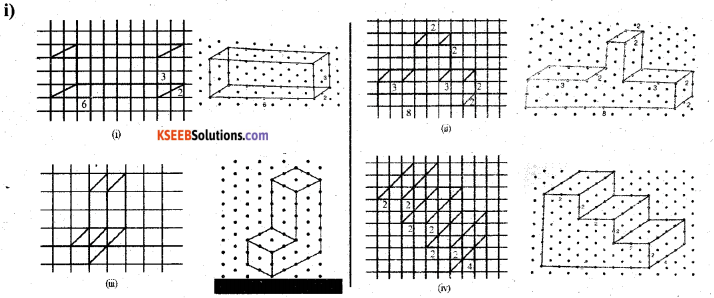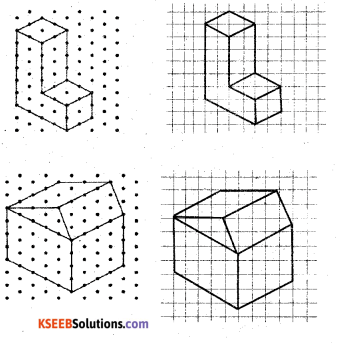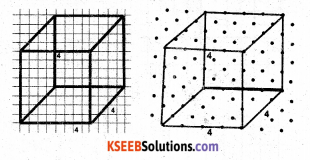# KSEEB Solutions for Class 7 Maths Chapter 15 Visualising Solid Shapes Ex 15.2

Students can Download Chapter 15 Visualising Solid Shapes Ex 15.2, Question and Answers, Notes Pdf, KSEEB Solutions for Class 7 Maths, Karnataka State Board Solutions help you to revise complete Syllabus and score more marks in your examinations.

## Karnataka State Syllabus Class 7 Maths Chapter 15 Visualising Solid Shapes Ex 15.2

Question 1.
Use isometric dot paper and make an isometric sketch for each one of the given shapes:
Solution:Question 2.
The dimensions of a cuboid are 5 cm, 3cm and 2 cm. Draw three different isometric sketches of this cuboid.
Solution:Question 3.
Three cubes each with 2 cm edge are placed side by side to form a cuboid. Sketch an oblique or isometric sketch of this cuboid.
Solution:Question 4.
Make an oblique sketch for each one of the given isometric shapes :
Solution:Question 5.
Give
(i) an oblique sketch and
(ii) an isometric sketch for each of the following :
a) A cuboid of dimensions 5 cm, 3 cm and 2 cm. (Is your sketch unique ?)
Solution:b) A cube with an edge 4 cm long.
Solution:error: Content is protected !!